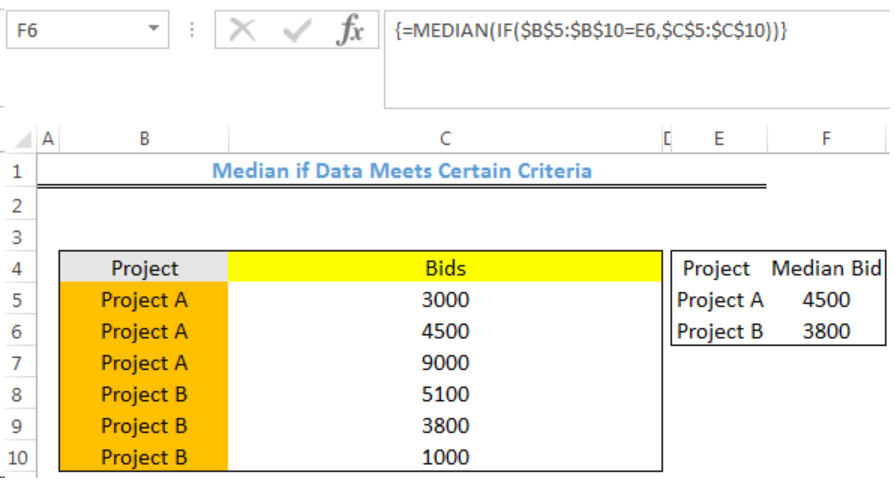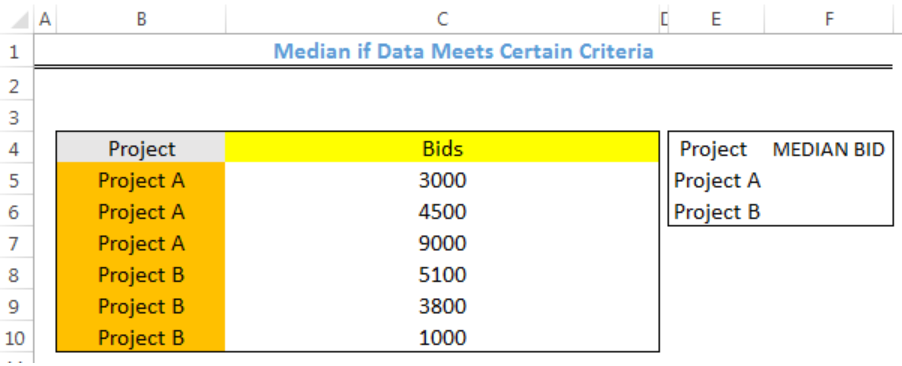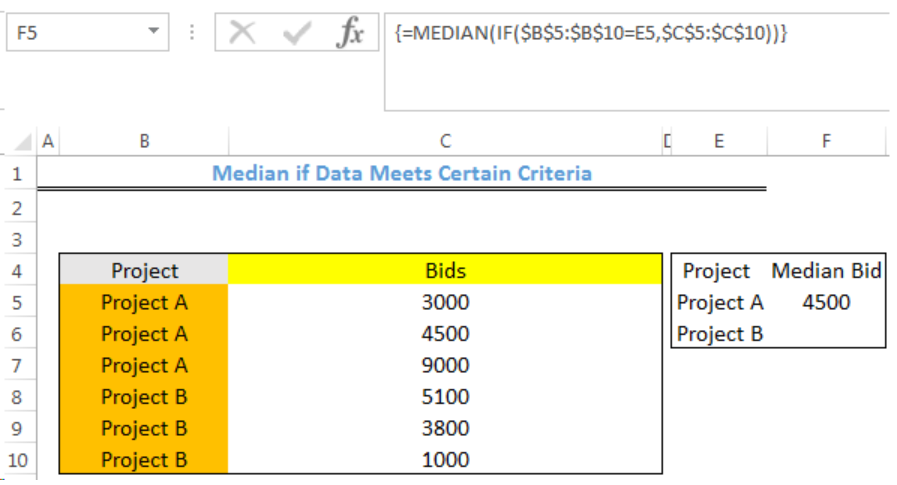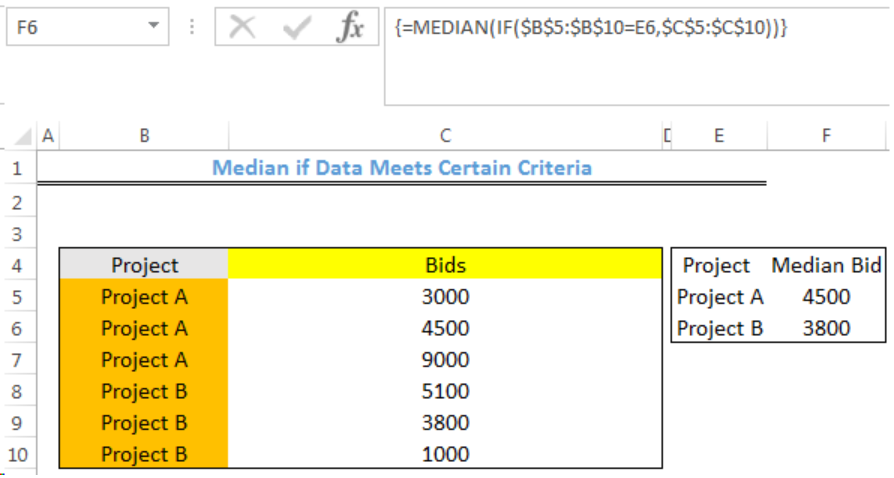Get instant live expert help with Excel or Google Sheets“My Excelchat expert helped me in less than 20 minutes, saving me what would have been 5 hours of work!”

#### Post your problem and you’ll get expert help in seconds.

Your message must be at least 40 characters
Our professional experts are available now. Your privacy is guaranteed.

# Learn How to Find a Median if Your Data Meets Certain Criteria

We can use a formula that combines the MEDIAN and IF functions to find the median of a set of data if the values meet a criteria. The steps below will walk through the process.Figure 1- How to Find the Median if Data Meets Certain Criteria

## Syntax

`=MEDIAN(IF(logical_test,value_if_true,value_if_false))`

• logical_test: This is the criteria that must be met for the median function to operate
• value_if_true: This is the returned result by the IF Function that tells the median function that the criteria is met and median function can operate
• value_if_false: This is the opposite of value_if_true

## Formula

`=MEDIAN(IF(\$B\$5:\$B\$10=\$E\$5,\$C\$5:\$C\$10))`

## Setting up the Data

Contractors have placed their bids for two separate projects in a company. The manager intends to find the median bid for Project A and Project B

• We will insert the Project names and Bids in Column B and Column C

• Our result will be returned in Column FFigure 2 – Setting up the Data

## Median if Data Meets Certain Criteria

• We will click on Cell F5
• We will insert the formula below into Cell F5
`=MEDIAN(IF(\$B\$5:\$B\$10=E5,\$C\$5:\$C\$10))`
• Because this is an array formula, we will press CTRL + SHIFT + ENTERFigure 3- Median if Data Meets Certain Criteria

• We will click on Cell F5 again
• We will double click on the fill handle tool which is the small plus sign you see at the bottom right of Cell F5. Select and drag down to copy the formula to Cell F6.Figure 4- Result of Median if Data Meets Certain Criteria

## Instant Connection to an Expert through our Excelchat Service

Most of the time, the problem you will need to solve will be more complex than a simple application of a formula or function. If you want to save hours of research and frustration, try our live Excelchat service! Our Excel Experts are available 24/7 to answer any Excel question you may have. We guarantee a connection within 30 seconds and a customized solution within 20 minutes.

Are you still looking for help with the Average function? View our comprehensive round-up of Average function tutorials here.

Solution exampleshello i need help creating a formula, if a value does not exsits put 0, if a value exist do nothing
Solved by M. F. in 9 minsCompute the weighted average of students score from a spreadsheet using different weight for each category of marks
Solved by G. E. in 20 minshi there, im making a spread sheet to track progress in a game and would like to create a drop log sheet, i was hoping to use the if function to detect weather a item is logged on a sheet then send the sheet number to a list if so
Solved by K. F. in 60 minsI have a schedule I'm doing with formulas to add workdays (across rows) to a start date column. I would like to write the equation like this: =ifError((workday(b2,1) = cell above, [value of cell above + 1] Help me form this correctly to get the (value of target cell) = (1 more than the value from the cell above) IF the target cell value started equal to the cell above.
Solved by M. J. in 20 minsI need a formula that will subtract the sum of one cell if the sum of another cell is greater than zero, and have it applied to cells across a document that is 30,000 entries and only in certain spots
Solved by F. J. in 20 minsGuest
Celeste Oddoye

Hello! I’m trying to find the median amount of time for how long it has taken for people to pick up packages from our front desk in the office. I have the data showing the times that packages were received, and the times when they were picked up, but I’m not sure how to find the median. Could you please help? What further information would you like me to provide, please?

Comment awaiting moderation